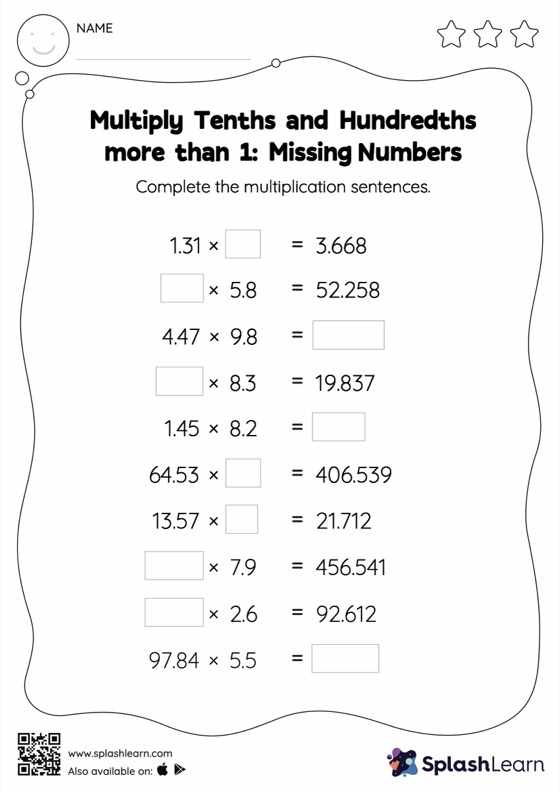# Multiply Tenths and Hundredths: 2-Digit Multiplication: Missing Numbers Worksheet

Home > Multiply Tenths and Hundredths: 2-Digit Multiplication: Missing NumbersThis worksheet consists of a set of questions on multiplication where students are required to multiply tenths and hundredths: 2-digit multiplication. As the worksheet uses a horizontal format, it allows for more creativity when it comes to how the student solves the problem. In contrast, in the vertical format, students usually employ the standard method to solve.